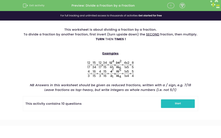# Divide a Fraction by another Fraction

In this worksheet, students will practise dividing a fraction by a fraction.Key stage:  KS 3

Curriculum topic:   Number

Curriculum subtopic:   Use Four Operations for All Numbers

Popular topics:   Fractions worksheets, Percentages worksheets

Difficulty level:#### Worksheet Overview

This activity is about dividing a fraction by a fraction.

To divide a fraction by another fraction, we can use the KFC method.

No, not the yummy chicken and chips method - but nearly as much fun!!

Keep the first fraction

Flip the second fraction

Change the ÷ to a ×

Once we have done this, we multiply the fractions as we normally would by multiplying the numerators, and multiplying the denominators.

Examples

 3 ÷ 1 = 3 x 4 = 12 5 4 5 1 5 4 ÷ 2 = 4 x 7 = 28 = 14 3 7 3 2 6 3

NB Answers in this worksheet should be given as reduced fractions.

Leave fractions as top-heavy, but write integers as whole numbers (i.e. 5 not 5/1)

Let's get started.

### What is EdPlace?

We're your National Curriculum aligned online education content provider helping each child succeed in English, maths and science from year 1 to GCSE. With an EdPlace account you’ll be able to track and measure progress, helping each child achieve their best. We build confidence and attainment by personalising each child’s learning at a level that suits them.

Get started